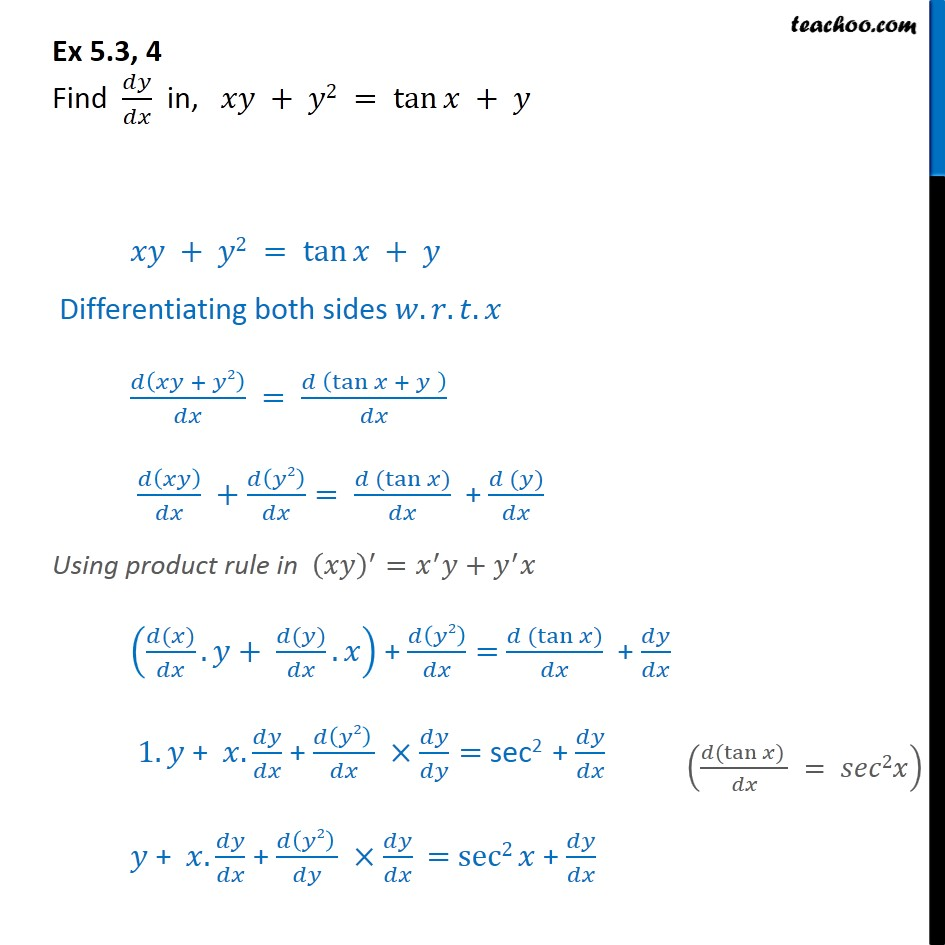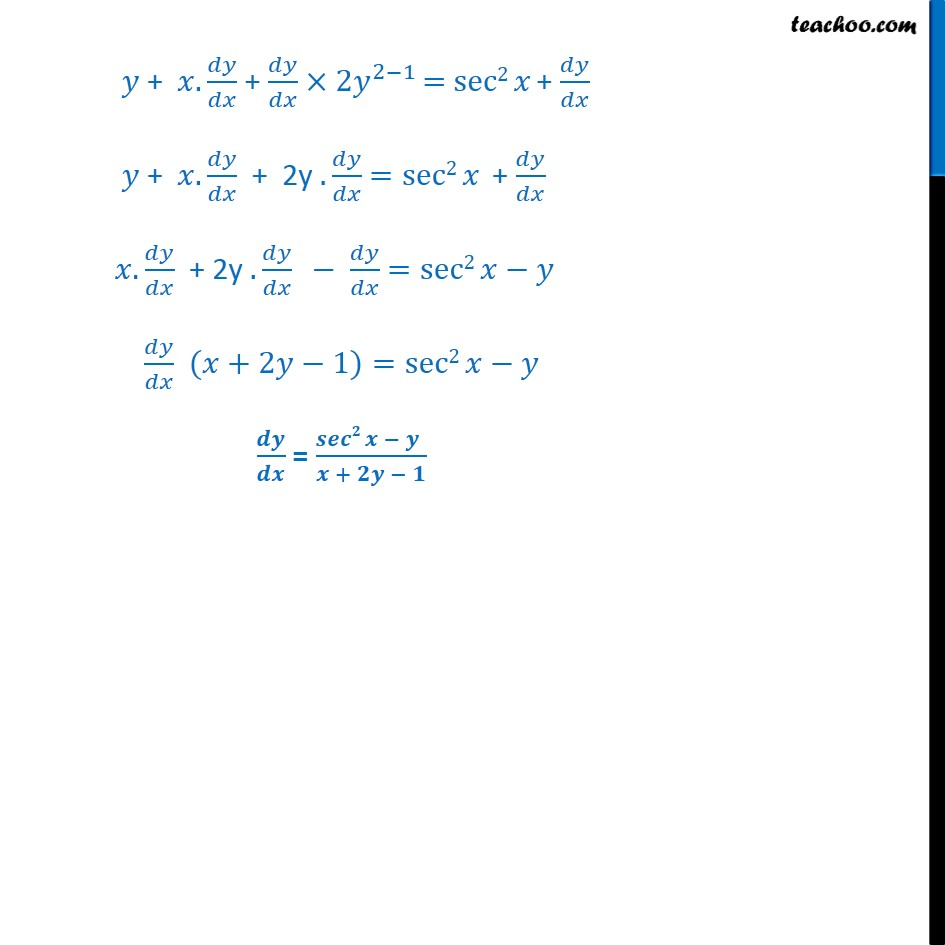1. Chapter 5 Class 12 Continuity and Differentiability
2. Concept wise
3. Finding derivative of Implicit functions

Transcript

Ex 5.3, 4 Find 𝑑𝑦﷮𝑑𝑥﷯ in, 𝑥𝑦 + 𝑦2 = tan⁡𝑥 + 𝑦 𝑥𝑦 + 𝑦2 = tan⁡𝑥 + 𝑦 Differentiating both sides 𝑤.𝑟.𝑡.𝑥 𝑑 𝑥𝑦 + 𝑦2﷯﷮𝑑𝑥﷯ = 𝑑 tan⁡𝑥 + 𝑦 ﷯﷮𝑑𝑥﷯ 𝑑 𝑥𝑦﷯﷮𝑑𝑥﷯ + 𝑑 𝑦2﷯﷮𝑑𝑥﷯= 𝑑 (tan⁡𝑥)﷮𝑑𝑥﷯ + 𝑑 (𝑦)﷮𝑑𝑥﷯ Using product rule in 𝑥𝑦﷯﷮′﷯= 𝑥﷮′﷯𝑦+ 𝑦﷮′﷯𝑥 𝑑(𝑥)﷮𝑑𝑥﷯. 𝑦+ 𝑑(𝑦)﷮𝑑𝑥﷯.𝑥﷯ + 𝑑 𝑦2﷯﷮𝑑𝑥﷯= 𝑑 (tan⁡𝑥)﷮𝑑𝑥﷯ + 𝑑𝑦﷮𝑑𝑥﷯ 1.𝑦 + 𝑥. 𝑑𝑦﷮𝑑𝑥﷯ + 𝑑 𝑦2﷯ ﷮𝑑𝑥﷯ × 𝑑𝑦﷮𝑑𝑦﷯= sec2 + 𝑑𝑦﷮𝑑𝑥﷯ 𝑦 + 𝑥. 𝑑𝑦﷮𝑑𝑥﷯ + 𝑑 𝑦2﷯ ﷮𝑑𝑦﷯ × 𝑑𝑦﷮𝑑𝑥﷯ = sec2 𝑥 + 𝑑𝑦﷮𝑑𝑥﷯ 𝑦 + 𝑥. 𝑑𝑦﷮𝑑𝑥﷯ + 𝑑𝑦﷮𝑑𝑥﷯× 2𝑦﷮2−1﷯ = sec2 𝑥 + 𝑑𝑦﷮𝑑𝑥﷯ 𝑦 + 𝑥. 𝑑𝑦﷮𝑑𝑥﷯ + 2y . 𝑑𝑦﷮𝑑𝑥﷯ =sec2 𝑥 + 𝑑𝑦﷮𝑑𝑥﷯ 𝑥. 𝑑𝑦﷮𝑑𝑥﷯ + 2y . 𝑑𝑦﷮𝑑𝑥﷯ − 𝑑𝑦﷮𝑑𝑥﷯ =sec2 𝑥−𝑦 𝑑𝑦﷮𝑑𝑥﷯ (𝑥+2𝑦−1)=sec2 𝑥−𝑦 𝒅𝒚﷮𝒅𝒙﷯ = 𝒔𝒆𝒄𝟐 𝒙 − 𝒚 ﷮𝒙 + 𝟐𝒚 − 𝟏﷯

Finding derivative of Implicit functions